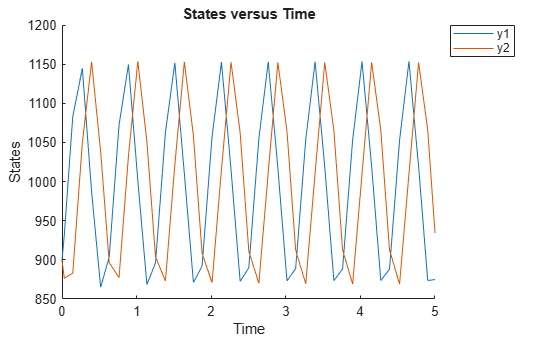# accelerate(SimFunction)

Prepare SimFunction object for accelerated simulations

## Syntax

```accelerate(F) ```

## Input Arguments

 `F` `SimFunction object` created by the `createSimFunction` method of a SimBiology model.

## Description

`accelerate(F)` prepares `SimFunction object` `F` for accelerated simulations.

Note

`F` is automatically accelerated at the first function execution. However, manually accelerate the object if you want it accelerated in your deployment applications.

## Examples

collapse all

`sbioloadproject lotka;`

Create a SimFunction object f with c1 and c2 as input parameters to be scanned, and y1 and y2 as the output of the function with no dose.

`f = createSimFunction(m1,{'Reaction1.c1', 'Reaction2.c2'},{'y1', 'y2'}, [])`
```f = SimFunction Parameters: Name Value Type ________________ _____ _____________ {'Reaction1.c1'} 10 {'parameter'} {'Reaction2.c2'} 0.01 {'parameter'} Observables: Name Type ______ ___________ {'y1'} {'species'} {'y2'} {'species'} Dosed: None ```

The SimFunction object f is not set for acceleration at the time of creation. But it will be automatically accelerated when executed.

`f.isAccelerated`
```ans = logical 0 ```

Define an input matrix that contains parameter values for c1 and c2.

`phi = [10 0.01];`

Run simulations until the stop time is 5 and plot the simulation results.

`sbioplot(f(phi,5));`Confirm the SimFunction object f was accelerated during execution.

`f.isAccelerated`
```ans = logical 1 ```